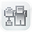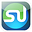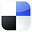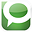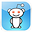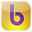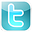Excel
STATISTICAL
Master

ANOVA - Analysis of Variance

Clear and Complete - WITH LOTS OF SOLVED PROBLEMS

# ANOVA  Independence Tests and Analysis of Variance

Lots of Worked-Out, Easy-To-Understand, Graduate-Level Problems  --->
( Scroll Down and Take a Look ! )

Introduction

ANOVA, Analysis of Variance, is a test to determine whether the means of several groups are equal. As such, ANOVA is often used to determine if three or more different methods or treatments have the same effect on a population. For example, ANOVA testing might be used to determine if three different teaching methods produce the same test scores with a group of students. The measured output must be some type of group average such as average test score per group or average sales per group. ANOVA testing might also be used to determine if different combinations of product pricing and promotion have different effects in different markets.

## Basic Description of ANOVA

In summary, ANOVA testing is used to judge whether three or more groups have the same mean (for example, same test scores) after each group has had a different treatment applied to it (for example, a different teaching method applied to each group). ANOVA - A Very Basic Definition

If there no real differences between the groups being tested, one would expect that any measured differences between the groups would not be much different than measured differences between samples taken from within individual groups.

A F Ratio ( sometimes called an F Statistic) compares the differences between groups to the differences within groups.

Conceptually, the F Ratio can be thought of as how different the means of groups are relative to the variability within the groups. It might also be helpful to view the following explanation:

 F Ratio = Real Differences + Random Differences Between Groups Random Differences Within Groups

The actual definition of the F Ratio is as follows:
 F Ratio = Variance of the Group Means Mean of the Within-Group Variances

This is sometimes shortened to:
 F Ratio = Mean Square Between Groups Mean Square Within-Group

The larger the value of the F Ratio (sometimes called the F Statistic), the greater the likelihood that the difference between groups is due to Real Differences and not just due to chance (Random Differences).

The required degree of certainty (for example, we want to be at least 95% that the groups are different) determines how large the F Ratio has to be for us to be able to state that the groups are different.

The distribution of the F Ratio is called the F Distribution. The F Distribution is a family of distributions, each described by the following two parameters:

ѵ1 = Degrees of Freedom Between Groups

ѵ2 = Degrees of Freedom Within Groups

Critical F Values have been calculated for various degrees of certainty (99% certainty, 95% certainty, etc.) for each of the basic F Distributions. The general rule use to state whether real differences exists between groups for a given level of certainty is as follows:

General Rule:

If F Statistic (ѵ1 , ѵ2) > F Critical (ѵ1 , ѵ2)----> The different treatments affected the output

If F Statistic (ѵ1 , ѵ2) < F Critical (ѵ1 , ѵ2)
----> The different treatments did not affect the output

The F Statistic and F Critical are calculated using the same ѵ1 and ѵ2. If the F Statistic is greater than the F Critical that is calculated for a specific degree of certainty, we can state that groups are statistically different.

ANOVA Tests the Null Hypothesis -

That Nothing Is Different Between Groups

The basic test of ANOVA is the Null Hypothesis that the different methods had no effect on the outcome that is being measured. Using the teaching method example, the Null Hypothesis in this case would be that the different teaching methods had no effect on the average test scores of student groups to which different
treatments (teaching methods) were applied.

The Null Hypotheses, which is being tested, states that the average test score from each of the three groups or populations to which a different teaching method was applied should be the same.

Required ANOVA  Assumptions

1) Normality - Each of the groups should be Normally distributed.

2) Independence of Treatments - No treatment of one group affects another.

3) Homoscedasticity - The groups have equal variances.

Homoscedasticity can be confirmed by a test called Levene's Test.

Normality can be confirmed by a number of tests, such Kolmogorov-Smirnov Test.

These tests are not discussed in this section.

Overview of ANOVA in Excel

The hand calculations for ANOVA are very tedious. Excel has a built-in ANOVA function that does a great job. The problem below is completed using hand-calculation but could much quicker using Excel's built-in ANOVA function. The completed problem below is the simplest type of ANOVA, known as Single Factor ANOVA.

Inside Excel's Data Analysis menu, there are three types of ANOVA analysis available:

1) Single Factor ANOVA

2) Two-Factor ANOVA Without Replication

3) Two-Factor ANOVA with Replication

Each ANOVA test type is explained below:

Single Factor ANOVA

Single Factor ANOVA tests the effect of just one factor, in this case, the teaching method, on the measured outputs. The measured outputs are the mean test scores for the groups that had the different teaching methods applied to them. The Null Hypothesis for this one factor states that varying that factor has no effect
on the outcome.

Two-Factor ANOVA Without Replication

Two-Factor ANOVA Without Replication - Allows testing of the original factor plus one other factor. For example, in addition to testing teaching methods, you could also test an additional factor, such as whether differences in teaching ability caused additional variation in the outcome of test average scores. Each factor has a Null Hypothesis which states that varying that factor had no effect on the outcome.

Two-Factor ANOVA With Replication

Two-Factor ANOVA With Replication allows for testing both factors as above. This method also allows us to test the effect of interaction between the factors upon the measured outcome. The test is replicated in two places. This allows for analysis of
whether the interaction between the two factors has an effect on the measured outcome. The Null Hypothesis for this interaction test states that varying the interaction between the two factors has no effect on the measured outcome. Each of the other two factors being tested also has its own Null Hypothesis.

Problem 1

Problem: Three Sales Closing Methods Tested With Single Factor ANOVA

Three different sale closing methods were used. Three groups of four salespeople were randomly chosen. Each group was instructed to use only one of the closing methods for all of their sales. Sales totals of each salesperson over the next two weeks were collected. Determine with a 95% level of certainty whether there is a difference in the effectiveness of the closing methods. Following are
sales results for all 12 salespeople:

 Sales Group 1 Closing Method 1 - Sales Salesperson 1 16 Salesperson 2 21 Salesperson 3 18 Salesperson 4 13

 Sales Group 2 Closing Method 2 - Sales Salesperson 5 19 Salesperson 6 20 Salesperson 7 21 Salesperson 8 20

 Sales Group 3 Closing Method 3 - Sales Salesperson 9 24 Salesperson 10 21 Salesperson 11 22 Salesperson 12 25

Problem Solving Steps

This is a Single Factor ANOVA test because we are testing only whether different variations of a single factor (Closing Method) have an effect on measured outcome (sales of each salesperson) using a different method. Nothing else is entered into the test that might have an effect on the measured outcome. The abilities of all salespeople are assumed to be similar. Each individual salesperson will use only one of the closing methods.

The Null Hypothesis for this test states that the closing methods used will have no effect on the measured output (sales).

Level of Certainty = 95% = 1 - α

Level of Significance = Alpha = α = 0.05

General Rule:

If F Statistic (ѵ1 , ѵ2) > F Critical (ѵ1 , ѵ2)----> The different treatments affected the output

If F Statistic (ѵ1 , ѵ2) < F Critical (ѵ1 , ѵ2)
----> The different treatments did not affect the output

Arrange the data as below to facilitate calculations:

 Sales Group --> Group 1 Group 2 Group 3 Treatment --> Method 1 Method 2 Method 3 Salesperson A 16 19 24 Salesperson B 21 20 21 Salesperson C 18 21 22 Salesperson D 13 20 25

 Column Total 68 80 92 Column Mean 17 20 23 Grand Mean = (17 + 20 + 23) / 3 Grand Mean = 20 Column Mean - Grand Mean -3 0 3 (Column Mean - Grand Mean)2 9 0 9 # Rows * [(Column Mean - Grand Mean)2] 36 0 36 Sum of Squares Between Groups = 36 + 0 + 36 = 72

 Method 1 Method 2 Method 3 16 19 24 21 20 21 18 21 22 13 20 25 68 80 92 68 / 4 = 80 / 4 = 92 / 4 = 17 20 23

 Method 1 Method 2 Method 3 16 - 17 19 - 20 24 - 23 21 - 17 20 - 20 21 - 23 18 - 17 21 - 20 22 - 23 13 - 17 20 - 20 25 - 23

 Method 1 Method 2 Method 3 -1 -1 1 4 0 -2 1 1 -1 -4 0 2

Square Each

 Method 1 Method 2 Method 3 1 1 1 16 0 4 1 1 1 16 0 4 34 2 10

Sum of Squares Within Treatments = 34 + 2 + 10 = 46

 Degrees of Freedom Between Groups DOF = # Groups - 1 = 3 - 1 = 2 Within Groups DOF = (# Columns) * (# Rows - 1) = 3 ( 4 - 1) = 9 Total Degrees of Freedom = 2 + 9 = 11

 Sum of Squares Between Groups Sum of Squares 72 Sum of Squares Within Groups 46 Total Sum of Squares 116

 Mean Squares MS = Mean Square = Sum of Squares / Degrees of Freedom SS df MS 72 2 36 46 9 5.1

 F Statistic(ѵ1 , ѵ2)  ---->  ѵ1 = DOF1 = 2, ѵ1 = DOF2 = 9 F Statistic (ѵ1=2,ѵ2=9) = (MS Between Groups) / (MS Within Groups) F Statistic (ѵ1=2,ѵ2=9) = 36 / 5.1 = 7.04

The calculated F Statistic(ѵ1 = 2, ѵ2 = 9) = 7.04. This is greater than F Criticalα=0.051 = 2, ѵ2 = 9) = 4.265. This indicates that there is less than a 5% chance that this result could have occurred if there was no difference in the effectiveness between the closing methods. Therefore, there is at least a 95% certainty that there is a real difference in effectiveness of the closing methods. The Null Hypothesis, which was therefore rejected, states that choice of closing methods does not affect sales.

If You Like This, Then Share It...